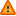Extracting_beta,_standard_error_and_confidence_interval_for_a_coordinate - MRC CBU Imaging WikiYou should trust both wikis because the password could be read by the particular administrators.

Clear message

# Extracting beta, standard error and confidence intervals for a coordinate

It is sometimes useful to pull out the parameter estimates and measures of dispersion from a location in the SPM results viewer. You could click through the GUI and get the data from the 'contrasts' struct that appears in your workspace (do plot > Contrast estimates and 90% CI. > Which contrast?), but this function is faster.

Do [beta,sterr,ci] = extractSPMData(xSPM,SPM) to get data for your current location. To pull data from another location (in mm), try [beta,sterr,ci] = extractSPMData(xSPM,SPM,[48 -67 28]).

All code is adapted from spm_graph.

```% This function extracts beta, st error and 90% CI
% for the voxel at the current location in the SPM
% results viewer (or at a location specified in
% the third argument).
% [beta,sterr,ci90] = extractSPMData(xSPM,SPM,[coords]);
% 11/6/2010 J Carlin
function [cbeta,SE,CI] = extractSPMData(xSPM,SPM,coords);

hReg = findobj('Tag','hReg'); % get results figure handle

% Use current coordinates, if none were provided
if ~exist('coords','var')
coords = spm_XYZreg('GetCoords',hReg);
end

% This is mostly pulled out of spm_graph
[xyz,i] = spm_XYZreg('NearestXYZ',coords,xSPM.XYZmm);
spm_XYZreg('SetCoords',xyz,hReg);
XYZ     = xSPM.XYZ(:,i); % coordinates

%-Parameter estimates:   beta = xX.pKX*xX.K*y;
%-Residual mean square: ResMS = sum(R.^2)/xX.trRV
%----------------------------------------------------------------------
beta  = spm_get_data(SPM.Vbeta, XYZ);
ResMS = spm_get_data(SPM.VResMS,XYZ);
Bcov  = ResMS*SPM.xX.Bcov;

CI    = 1.6449;
% compute contrast of parameter estimates and 90% C.I.
%------------------------------------------------------------------
Ic = xSPM.Ic; % Use current contrast
cbeta = SPM.xCon(Ic).c'*beta;
SE    = sqrt(diag(SPM.xCon(Ic).c'*Bcov*SPM.xCon(Ic).c));
CI    = CI*SE;```